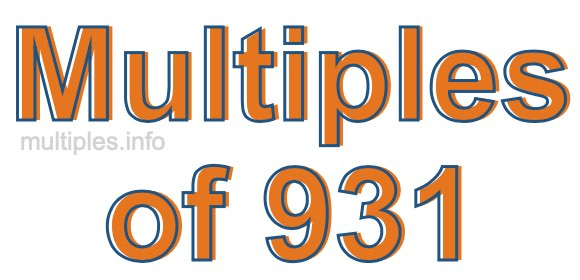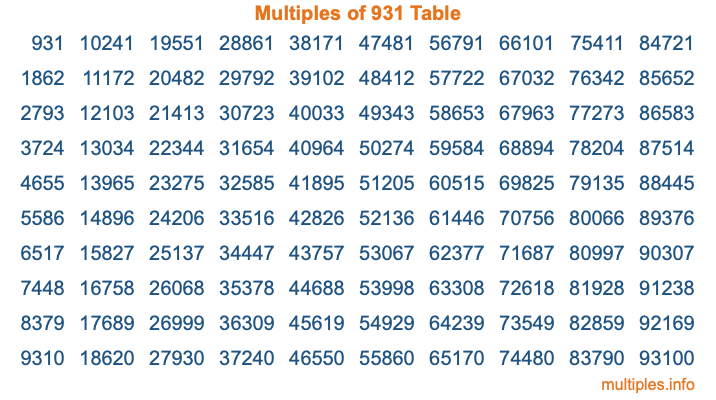Multiples of 931Welcome to the Multiples of 931 page. Here we will first teach you everything you will ever need to know about the multiples of 931, and then give you a study guide summary of everything we taught you to make sure you remember it all. Use this page to look up facts and learn information about the multiples of 931. This page will make you a multiples of nine hundred thirty-one expert!

Definition of Multiples of 931
Multiples of 931 are all the numbers that when divided by 931 equal an integer. Each of the multiples of 931 are called a multiple. A multiple of 931 is created by multiplying 931 by an integer.

Therefore, to create a list of multiples of 931, you start with 1 multiplied by 931, then 2 multiplied by 931, then 3 multiplied by 931, and so on for as long as you want. Thus, the list of the first five multiples of 931 is 931, 1862, 2793, 3724, and 4655. To see a larger list of multiples of 931, see the printable image of Multiples of 931 further down on this page. We also have a category where you can choose any nth multiple of 931.

Multiples of 931 Checker
The Multiples of 931 Checker below checks to see if any number of your choice is a multiple of 931. In other words, it checks to see if there is any number (integer) that when multiplied by 931 will equal your number. To do that, we divide your number by 931. If the the quotient is an integer, then your number is a multiple of 931.

Is  a multiple of 931?

Least Common Multiple of 931 and ...
A Least Common Multiple (LCM) is the lowest multiple that two or more numbers have in common. This is also called the smallest common multiple or lowest common multiple and is useful to know when you are adding our subtracting fractions. Enter one or more numbers below (931 is already entered) to find the LCM.

Check out our LCM Calculator if you need more details about the Least Common Multiple or if you need the LCM for different numbers for adding and subtraction fractions.

nth Multiple of 931
As we stated above, 931 is the first multiple of 931, 1862 is the second multiple of 931, 2793 is the third multiple of 931, and so on. Enter a number below to find the nth multiple of 931.

th multiple of 931

Multiples of 931 vs Factors of 931
931 is a multiple of 931 and a factor of 931, but that is where the similarities end. All postive multiples of 931 are 931 or greater than 931. All positive factors of 931 are 931 or less than 931.

Below is the beginning list of multiples of 931 and the factors of 931 so you can compare:

Multiples of 931: 931, 1862, 2793, 3724, 4655, etc.

Factors of 931: 1, 7, 19, 49, 133, 931

As you can see, the multiples of 931 are all the numbers that you can divide by 931 to get a whole number. The factors of 931, on the other hand, are all the whole numbers that you can multiply by another whole number to get 931.

It's also interesting to note that if a number (x) is a factor of 931, then 931 will also be a multiple of that number (x).

Multiples of 931 vs Divisors of 931
The divisors of 931 are all the integers that 931 can be divided by evenly. Below is a list of the divisors of 931.

Divisors of 931: 1, 7, 19, 49, 133, 931

The interesting thing to note here is that if you take any multiple of 931 and divide it by a divisor of 931, you will see that the quotient is an integer.

Multiples of 931 Table
Below is an image of the first 100 multiples of 931 in a table. The table is in chronological order, column by column. The first column has the first ten multiples of 931, the second column has the next ten multiples of 931, and so on.The Multiples of 931 Table is also referred to as the 931 Times Table or Times Table of 931. You are welcome to print out our table for your studies.

Negative Multiples of 931
Although not often discussed or needed in math, it is worth mentioning that you can make a list of negative multiples of 931 by multiplying 931 by -1, then by -2, then by -3, and so on, to get the following list of negative multiples of 931:

-931, -1862, -2793, -3724, -4655, etc.

Multiples of 931 Summary
Below is a summary of important Multiples of 931 facts that we have discussed on this page. To retain the knowledge on this page, we recommend that you read through the summary and explain to yourself or a study partner why they hold true.

There are an infinite number of multiples of 931.

A multiple of 931 divided by 931 will equal a whole number.

931 divided by a factor of 931 equals a divisor of 931.

The nth multiple of 931 is n times 931.

The largest factor of 931 is equal to the first positive multiple of 931.

931 is a multiple of every factor of 931.

931 is a multiple of 931.

A multiple of 931 divided by a divisor of 931 equals an integer.

931 divided by a divisor of 931 equals a factor of 931.

Any integer times 931 will equal a multiple of 931.

Multiples of a Number
Here you can get the multiples of another number, all with the same attention to detail as we did for multiples of 931 on this page.

Multiples of
Multiples of 932
Did you find our page about multiples of nine hundred thirty-one educational? Do you want more knowledge? Check out the multiples of the next number on our list!